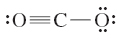# Problem: How important is the resonance structure shown here to the overall structure of carbon dioxide?

###### FREE Expert Solution

We are being asked to determine how important the given resonance structure is to the overall structure of carbon dioxide.

Recall that Resonance Structures are used to represent the different possible bonding arrangements in a molecule.

To determine the importance of the given Lewis Structure of CO2, we need to do the following steps:

Step 1: Determine the central atom in the molecule.

Step 2: Calculate the total number of valence electrons present.

Step 3: Demonstrate whether given Lewis structure for COthe satisfies octet rule

For CO2:

Step 1: Carbon (EN = 2.5) is less electronegative than oxygen (EN = 3.5) so carbon is the central atom.

Step 2: The total number of valence electrons present in CO2 is:

Group             Valence Electrons

C         4A                   1 × 4 e4 e

O         6A                   2 × 6 e12 e

Total: 16 valence e

Step 3: Draw the Lewis Structure for CO2

80% (448 ratings)###### Problem Details

How important is the resonance structure shown here to the overall structure of carbon dioxide?